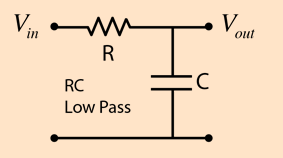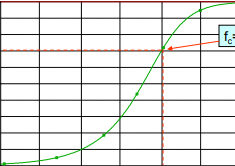# RC and RL Low Pass Filter

0
231

## RC and RL Low Pass Filter

### TYPES OF LOW PASS FILTER

#### As we know that low pass filter allows only low frequencies upto cut-off frequency, fc. The range of frequency upto fc is called passband of the filter.  A simple low pass RC filter is shown in the figure below:Fig-1 :low pass RC circuit

#### * Cutoff Frequency  fc = 1/ 2*π*C*R * Output Voltage, Vo = Vi* {(-j)*Xc / (R-jXc)* At the cutoff frequency , output signal voltage is reduced to 70.7% of the input voltage i.e, output voltage ,Vo = 70.7% * Vi * The output is -3 dB at cutoff frequency.* The phase angle between output voltage Vo and input voltage Vi is -45 at cutoff frequency.* R = Xc , at cutoff frequencyFrequency Response of Low Pass RC Filter This figure shows the frequency response curve of such filter. It shows that how the Output Voltage ,Vo varies with the signal frequency .Fig-2 :Frequency Response of Low pass RC circuit

### LOW-PASS RL FILTER

#### As we know that low pass filter allows only low frequencies upto cut-off frequency, fc. The range of frequency upto fc is called passband of the filter.

A simple low pass RL filter is shown in the figure below:Fig-3 :low pass RL circuit

The output voltage is taken across the resistance. The reactance offered by inductor L increases with the increase in frequency, therefore when the frequency is low then reactance offered by inductor is low. Hence, low frequencies upto fc can pass through coil without much opposition

* Cutoff Frequency, fc = L/ 2*π*R

* Output Voltage, Vo = Vi* {R / (R+jXL

* At the cutoff frequency , output signal voltage is reduced to 70.7% of the input voltage i.e, output voltage ,Vo = 70.7% * Vi = 0.707* Vi

* The output is -3 dB at cutoff frequency.

* The phase angle between output voltage Vo and input voltage Vi is +45 at cutoff frequency.

R = XL , at cutoff frequency

#### Frequency Response of Low Pass RL Filter This figure shows the frequency response curve of low pass RL filter. It shows that how the Output Voltage ,Vo varies with the signal frequency .Fig- 4 :Frequency Response of low pass RL filter

READ HERE  Asynchronous Motor – Type, Features, How does it Works